# Algebraic Expression Lesson Plan For B.Ed And Mathematics Teachers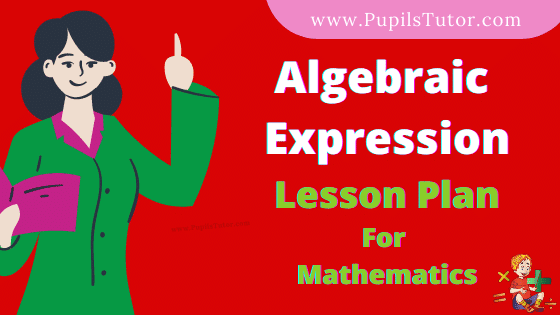Hello Friends, Welcome To Our Website. How Are You? Hope You Are Doing Well,

If You Are Searching For Algebraic Expression Lesson Plan Then You Have Come To The Right Place. Here We Have Shared The Lesson Plan On Algebraic Expressions For B.Ed, D.El.Ed, And School Teachers.

This Mega Teaching Mathematics Lesson Plan On Algebraic Expression Is Specially Made For The B.Ed 1st And 2nd Year Students But All The School Teachers And  Trainee Teacher Of All Classes Can Prepare Their Final Teaching Plan Very Easily With The Help Of This Model Algebraic Expression Lesson Plan For Class 6 To 9.

Brief Overview Of The Lesson Plan

 Class 6,7 And 8 Topic Algebraic Expression Subject Math Lesson Plan Type Mega Teaching Skill Real School Teaching

The Topics And Points That Are Covered In This Maths Lesson Plan On Algebraic Expression Are : What Is Algebraic Expression,  Algebraic Identity, Solving The Questions Using Formula With Examples, Practice Questions…....

## Algebraic Expression Lesson Plan –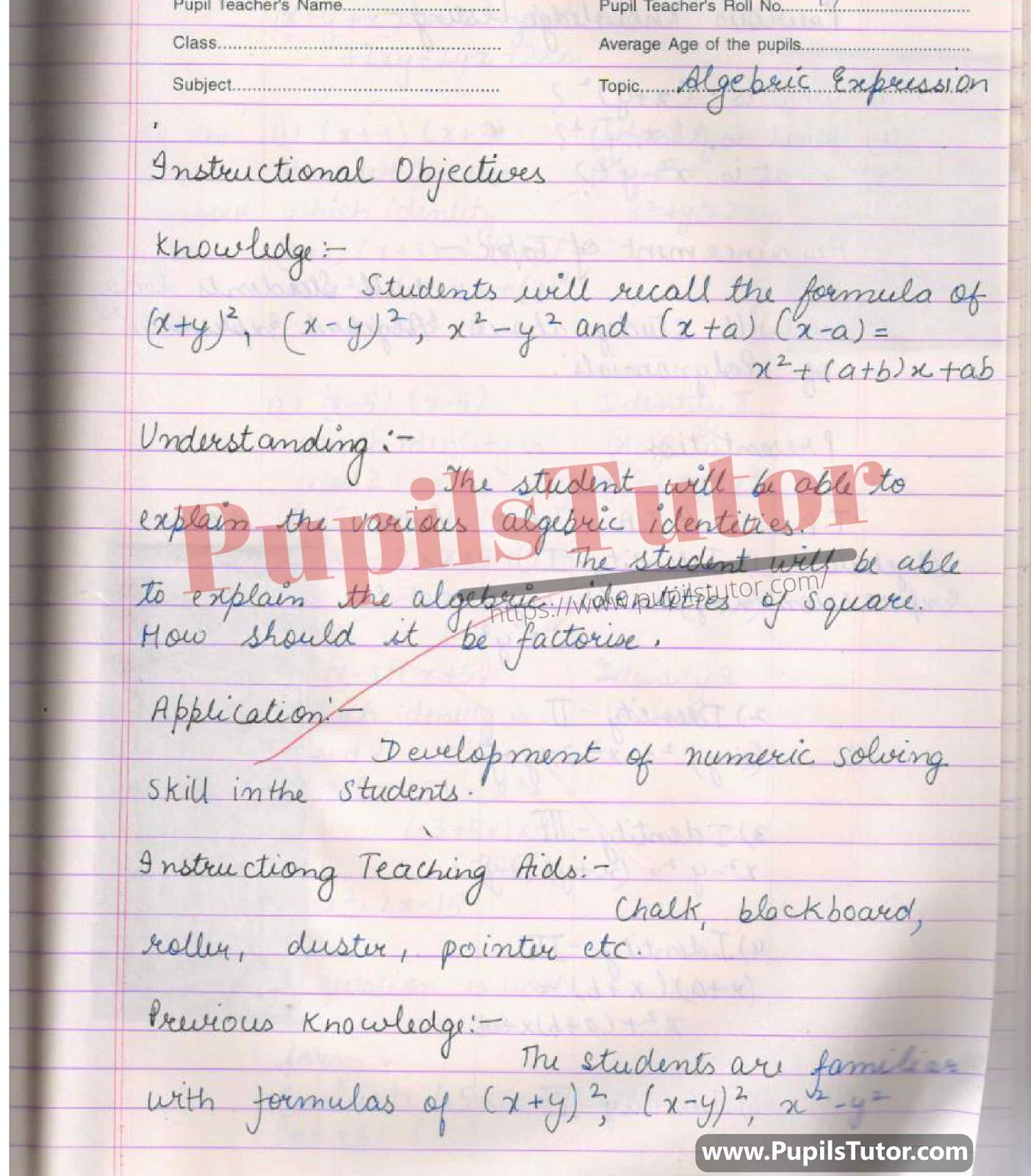## Mathematics Mega Teaching Algebraic Identity, Algebraic Expression, Algebra Lesson Plan For B.Ed And Deled In English Free Download PDF And PPT –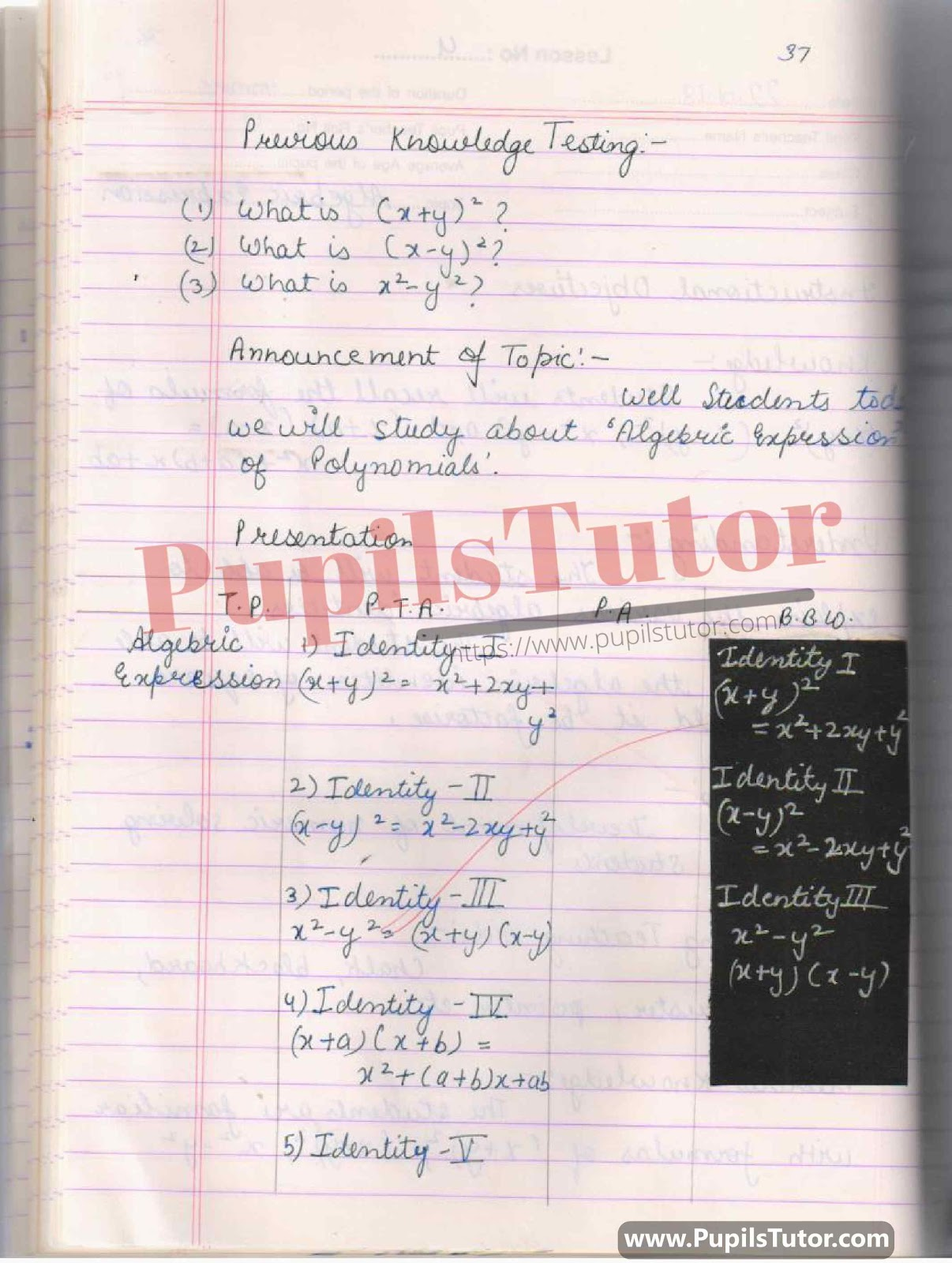## Math Lesson Plan On Algebra, Algebraic Expression For Class/Grade 6,7 And 8 For CBSE NCERT School, College Teachers And All Mathematics Students –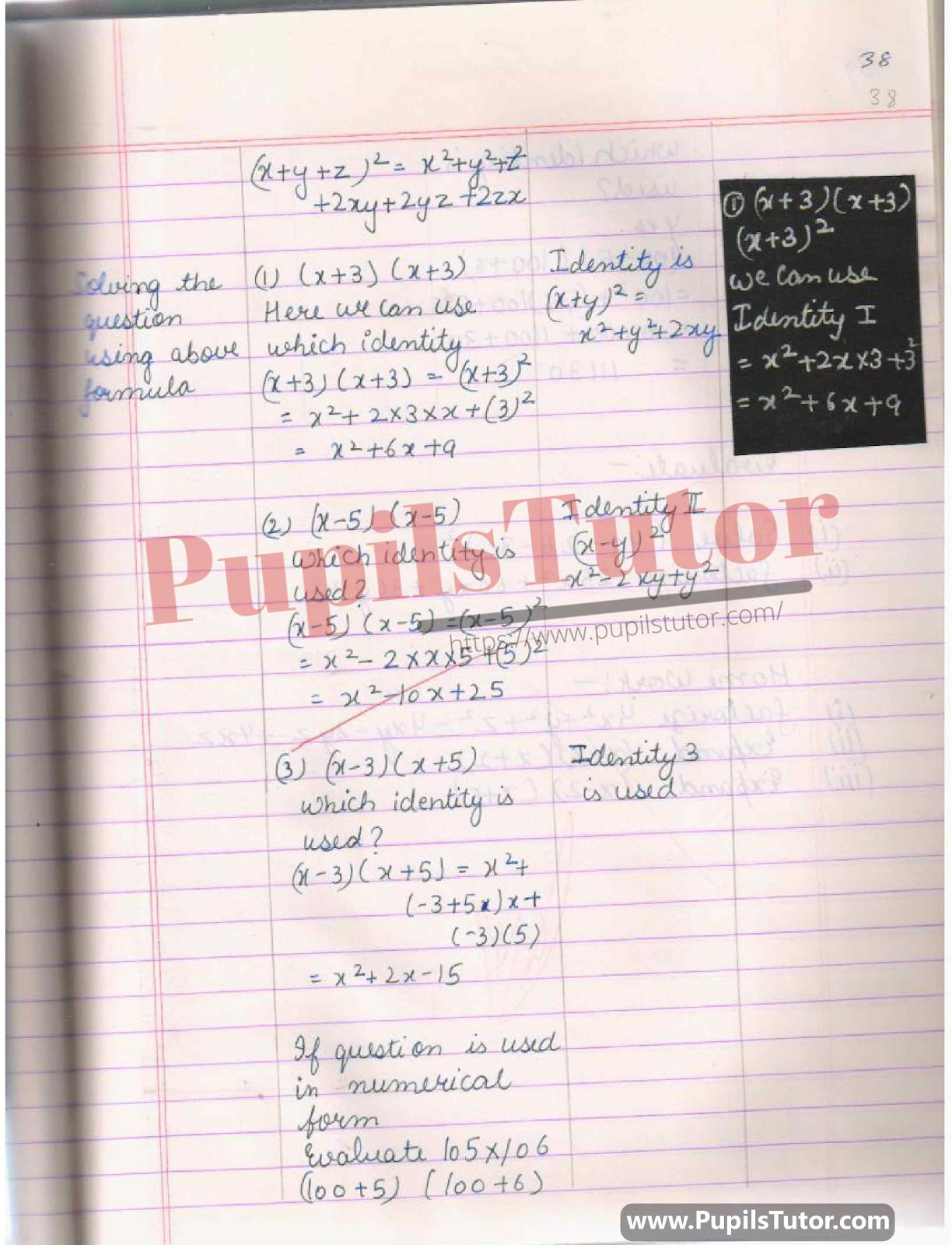## BED, DELED, BTC, BSTC, M.ED, DED And NIOS Teaching Of Maths Innovative Digital Lesson Plan Format On Algebraic Expressions, Algebraic Identities Topic For Class 4th 5th 6th 7th 8th 9th, 10th–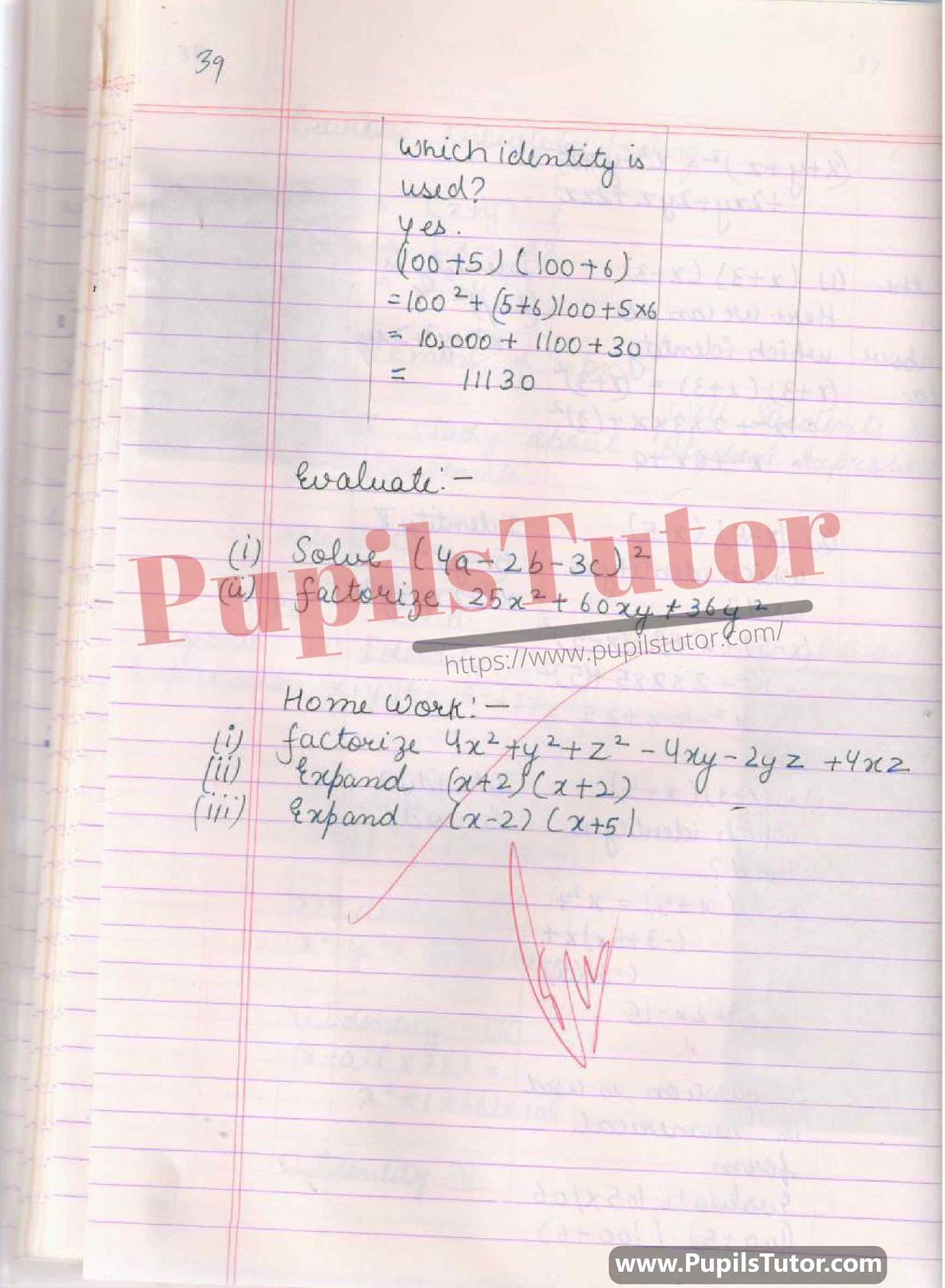You Might Also Like:

Further Reference

 B.Ed. Question Bank And Exam Notes A Complete Guide For B.ED. Entrance Examination CTET & TETs Preparation Material Teaching Aptitude and Attitude Test Book UP B.Ed Guidebook & Latest Practice Sets Book For Entrance Exam B.Ed CET Delhi University B.Ed Entrance Test Latest Practice Sets IGNOU B.Ed. Entrance Test: Previous Years Papers (Solved) Assignments And Files Lesson Planning Files And Guide

On Our Website www.PupilsTutor.Com, We Have Also Shared Lots Of Other Lesson Plans Of All Subjects On Various Teaching Skills Like Micro-Teaching, Mega Teaching, Discussion Skill, Real School Teaching And Practice Skill, Simulated And Observation Skills. You Can Also Check Them.

If You Liked This Algebraic Expression Lesson Plan For Class 6,7 And 8 Then Please Share Our Efforts With Your Friends Also.

You Can Also Share Your Lesson Plans, Assignments, Files, Papers And Study Notes With Us To Help Other Students .

#### List Of Some More Mathematics Lesson Plans

 Profit And Loss Lesson Plan For Math Sum Of The Angles Of A Triangle Lesson Plan For Math Trigonometry Ratio Lesson Plan For Math Circle Lesson Plan For Math Properties Of Angle In Circle Lesson Plan For Math Pie Chart Lesson Plan For Math Three Dimensional Shape Lesson Plan For Math Trigonometry Lesson Plan For Math Parts Of A Circle Lesson Plan For Math Compound Interest Lesson Plan For Math Volume Of Cone And Cylinder Lesson Plan For Math Area Related To Circle Lesson Plan For Math Integer Lesson Plan For Math Surface Area Of Cone And Cylinder Lesson Plan For Math Statistics And Arithmetic Mean Lesson Plan For Math Volume Of Right Circular Cylinder Lesson Plan For Math Circular Trigonometry Function Lesson Plan For Math 3 D Shapes Lesson Plan For Math Coordinate Geometry Section Formula Lesson Plan For Math Volume And Surface Area Of Sphere Lesson Plan For Math Theorem Lesson Plan For Math Curved And Total Surface Area Of Cylinder Lesson Plan For Math Quadrilateral Lesson Plan For Math Height Distance Lesson Plan For Math Curved And Total Surface Area Of Cone Lesson Plan For Math Coordinate Geometry Lesson Plan For Math Similar Triangle Lesson Plan For Math Polynomial Lesson Plan For Math Trigonometry Transformation Formula Lesson Plan For Math Surface Area Of Cube And Cuboid Lesson Plan For Math Algebraic Expression Lesson Plan For Math Proportion Lesson Plan For Math